Related Articles

# Create Multiple Buttons in Matplotlib

• Last Updated : 15 Mar, 2021

We all very well know that Python offers us a very beautiful library named Matplotlib that has the support of numerous methods using which we can plot graphs and visualize our data. Many a time, we all want our plots to look appealing and become interactive at the same time. For that purpose, buttons can be added in our matplotlib plots that can be used to trigger some on-click events and make our plots interactive.

In this article, we will be reading about creating multiple buttons in Matplotlib using the Buttons widget. We will use these buttons to create certain events (here plotting different graphs) when they get clicked. Initially, we will be plotting a graph for y=x and then make three different buttons named Prev, Next, and Home.

Attention geek! Strengthen your foundations with the Python Programming Foundation Course and learn the basics.

To begin with, your interview preparations Enhance your Data Structures concepts with the Python DS Course. And to begin with your Machine Learning Journey, join the Machine Learning - Basic Level Course

• When the Home button will be clicked, the initial page where a graph for y = x will be shown.
• When the Prev button will be clicked, a graph for y=will be shown.
• When the Next button will be clicked, a graph for y = 2x will be shown.

## Python

 `import` `numpy as np``import` `matplotlib.pyplot as plt``from` `matplotlib.widgets ``import` `Button`` ` ` ` `# Generating x and y-values``x ``=` `np.arange(``0``, ``1``, ``0.02``)``y ``=` `x`` ` `fig, ax ``=` `plt.subplots()``plt.subplots_adjust(left``=``0.1``, bottom``=``0.3``)``p, ``=` `plt.plot(x, y, color``=``'red'``)``ax.title.set_text(``'Graph for y = x'``)`` ` `# Home button``axButn1 ``=` `plt.axes([``0.1``, ``0.1``, ``0.1``, ``0.1``])``btn1 ``=` `Button(``  ``axButn1, label``=``"Home"``, color``=``'pink'``, hovercolor``=``'tomato'``)`` ` `# To plot a graph for y = x``def` `plot1(event):``    ``p.set_xdata(x)``    ``p.set_ydata(x)``    ``ax.title.set_text(``'Graph for y = x'``)``    ``plt.draw()`` ` ` ` `btn1.on_clicked(plot1)`` ` `# Previous button``axButn2 ``=` `plt.axes([``0.3``, ``0.1``, ``0.1``, ``0.1``])``btn2 ``=` `Button(``  ``axButn2, label``=``"Prev"``, color``=``'pink'``, hovercolor``=``'tomato'``)`` ` `# To plot a graph for y = x**2``def` `plot2(event):``    ``p.set_xdata(x)``    ``p.set_ydata(x``*``*``2``)``    ``ax.title.set_text(``'Graph for y = x**2'``)``    ``plt.draw()`` ` ` ` `btn2.on_clicked(plot2)`` ` `# Next button``axButn3 ``=` `plt.axes([``0.5``, ``0.1``, ``0.1``, ``0.1``])``btn3 ``=` `Button(``  ``axButn3, label``=``"Next"``, color``=``'pink'``, hovercolor``=``'tomato'``)`` ` `# To plot a graph for y = 2x``def` `plot3(event):``    ``p.set_xdata(x)``    ``p.set_ydata(``2``*``x)``    ``ax.title.set_text(``'Graph for y = 2x'``)``    ``plt.draw()`` ` `btn3.on_clicked(plot3)``plt.show()`

As stated earlier, initially a graph will be plotted for y=x, for that, a list of x-values in the range of 0 to 1 using np.arange() function will be generated and then assigned the same values to y-variable as well and then made a subplot to plot this graph. To note, plt.subplots_adjust() function is used to position the plot, the left and bottom parameter set the padding from the left side and the bottom of the graph.  The initial graph will be displayed as: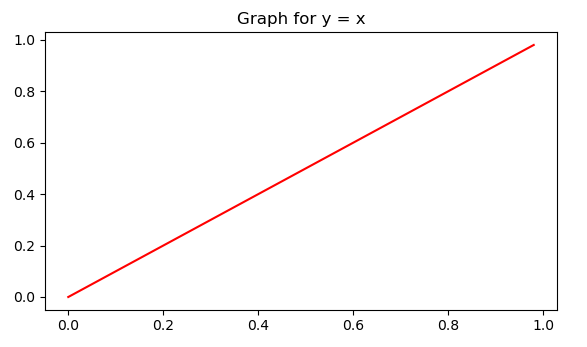Now that we have plotted our initial graph, let’s start adding our buttons and proceed to the methodology to add the Home button and in the same manner other two can be added.

The plt.axes() function accepts a list as an argument denoting the attributes of the button related to its position and size. The four elements inside the list corresponding to the left, bottom, width, and height values respectively.  Now, we will be using the Button module from matplotlib.widgets library to make our button. The first argument it takes is the attributes of the button, apart from that the label parameter takes the name to be shown on the button, the color parameter decides the color of the button and the hovercolor parameter shows the changes in the color of the button when we hover our mouse pointer over the button (here it changes from the pink color to color of a tomato that is red).

We have now made the button, but to make some events happen when they get clicked, we need to create some on-click events. It can be done using the on_clicked() method of the button. The on_clicked() method accepts a custom function as a parameter that describes what action will be performed when the button gets clicked.  For the Home button, plot1 is made as to the custom function which displays the graph of y=x which will be shown as: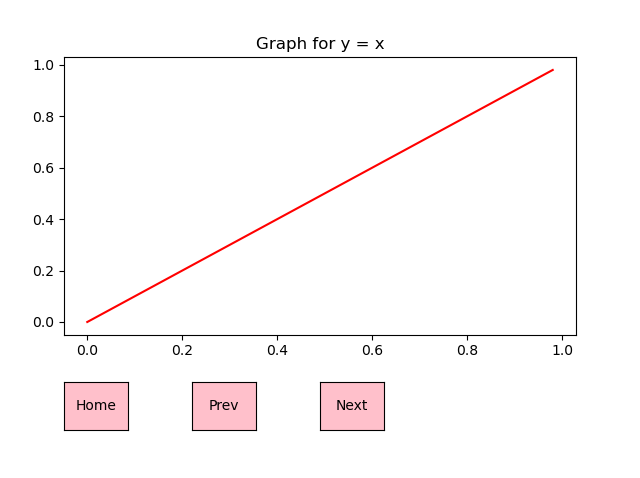Similarly, the Prev button can also be made to display the graph for y =when they get clicked, and it will be shown as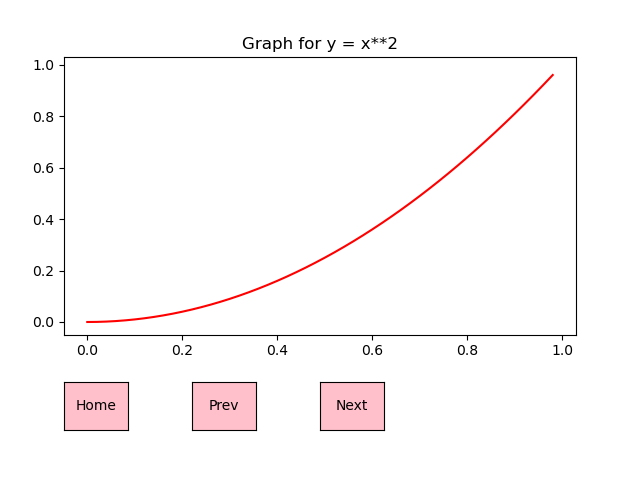Similarly, the Next button is used to display the graph for y = 2x when they get clicked, and it will be shown as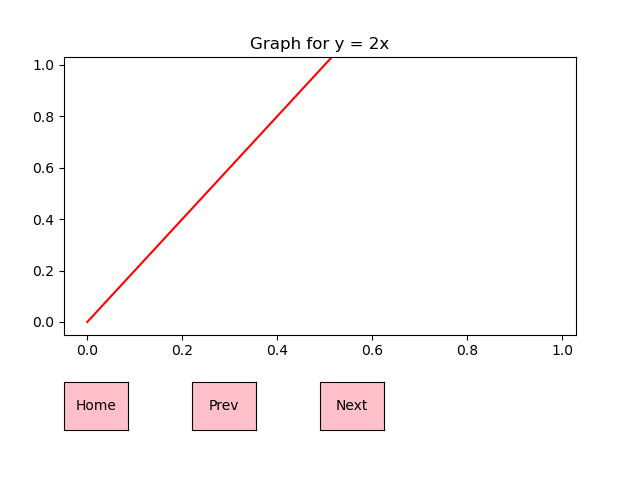Output: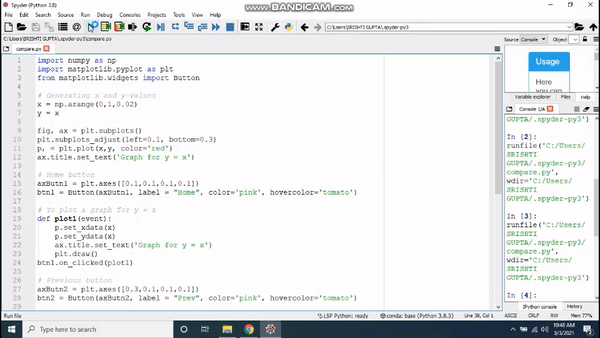My Personal Notes arrow_drop_up